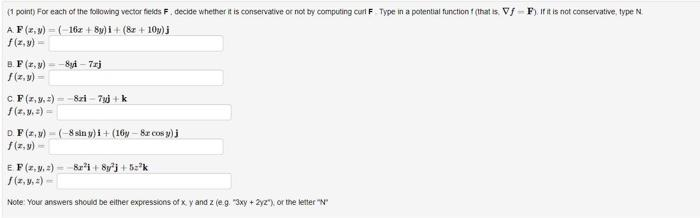### Create an Account

Already have account?

### Forgot Your Password ?

Home / Questions / 1 point) For each of the following vector fields F. decide whether it is conservative or n...

# 1 point) For each of the following vector fields F. decide whether it is conservative or not by computing curt F Type in a potential function f (that ts. Vf F if it is not conservative, type N. AF(x,y

1 point) For each of the following vector fields F. decide whether it is conservative or not by computing curt F Type in a potential function f (that ts. Vf F if it is not conservative, type N. AF(x,y)=( 16x + 8y)i + (8 + 10) f(x,y) B. F(x,y)=-By-7j F(x,y) - CF(x,y,z) = 8zi - 7+k f(x,y) = DF(x,y) = (-8 siny)i + (16y- &r cosy) f(x,y) EF (3,3,3) Br1+By+bak f(x,y,z) - Note: Your answers should be either expressions of xy and leg.3xy + 232") or the letterApr 13 2021 View more View Less

#### Answer (Solved)Subscribe To Get Solution